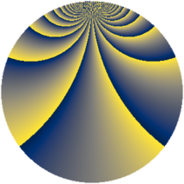# Properties

 Label 1840.2.cvLevel $1840$ Weight $2$ Character orbit 1840.cv Rep. character $\chi_{1840}(101,\cdot)$ Character field $\Q(\zeta_{44})$ Dimension $3840$ Sturm bound $576$

# Related objects

## Defining parameters

 Level: $$N$$ $$=$$ $$1840 = 2^{4} \cdot 5 \cdot 23$$ Weight: $$k$$ $$=$$ $$2$$ Character orbit: $$[\chi]$$ $$=$$ 1840.cv (of order $$44$$ and degree $$20$$) Character conductor: $$\operatorname{cond}(\chi)$$ $$=$$ $$368$$ Character field: $$\Q(\zeta_{44})$$ Sturm bound: $$576$$

## Dimensions

The following table gives the dimensions of various subspaces of $$M_{2}(1840, [\chi])$$.

Total New Old
Modular forms 5840 3840 2000
Cusp forms 5680 3840 1840
Eisenstein series 160 0 160

## Trace form

 $$3840q + 8q^{4} + 12q^{6} + O(q^{10})$$ $$3840q + 8q^{4} + 12q^{6} - 8q^{10} + 16q^{11} + 12q^{12} + 8q^{14} + 16q^{15} - 32q^{16} - 40q^{18} + 16q^{19} - 8q^{22} - 8q^{24} - 24q^{27} - 40q^{28} + 32q^{29} - 36q^{34} + 20q^{36} + 32q^{37} + 180q^{38} - 80q^{42} - 16q^{43} - 8q^{44} + 356q^{46} + 80q^{48} + 384q^{49} - 36q^{50} - 16q^{51} - 64q^{52} - 32q^{53} + 144q^{54} - 88q^{56} - 216q^{58} + 24q^{59} - 32q^{61} - 60q^{62} - 80q^{63} + 44q^{64} + 80q^{66} - 80q^{67} + 96q^{68} - 16q^{69} + 116q^{72} + 104q^{74} + 144q^{77} + 92q^{78} - 32q^{79} + 384q^{81} + 36q^{82} + 96q^{84} + 32q^{85} - 72q^{86} + 40q^{88} + 52q^{92} - 480q^{93} + 120q^{94} - 136q^{96} - 64q^{98} + 80q^{99} + O(q^{100})$$

## Decomposition of $$S_{2}^{\mathrm{new}}(1840, [\chi])$$ into newform subspaces

The newforms in this space have not yet been added to the LMFDB.

## Decomposition of $$S_{2}^{\mathrm{old}}(1840, [\chi])$$ into lower level spaces

$$S_{2}^{\mathrm{old}}(1840, [\chi]) \cong$$ $$S_{2}^{\mathrm{new}}(368, [\chi])$$$$^{\oplus 2}$$